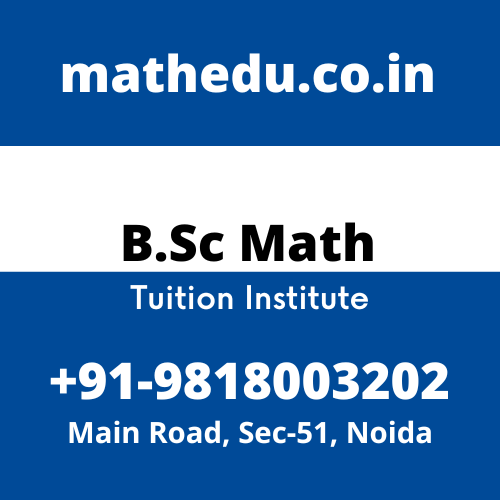# Differential Equations Tuition Class In Noida

### Differential Equations Tuition Class In NoidaAre You Looking for Differential Equations Tuition Class In Noida? Math Education Is a Live Tutoring Sites for The Best B.Sc Mathematics Tuition In Noida, Engineering Diploma, BBA, BCom. Highly Qualified Faculties Available to Teach University Students with Mathematics Subjects. Call Now +91-9818003202 For the  Differential Equations Tuition Class In Noida.

## Course Objectives

The main objectives of this course are to introduce the students to the exciting world of Differential Equations, Mathematical Modeling and their applications. Hence, Join the best Differential Equations Tuition Class In Noida

### Course Learning Outcomes: The course will enable the students to:

i) Formulate Differential Equations for various Mathematical models.
ii) Solve first order non-linear differential equation and linear differential equations of higher order using various techniques.
iii) Apply these techniques to solve and analyze various mathematical models

## Differential Equations and Mathematical Modeling Tuition

Differential equations and mathematical models, Order and degree of a differential equation, Exact differential equations and integrating factors of first order differential equations, Reducible second order differential equations, Application of first order differential equations to equations to acceleration-velocity model, Growth and decay model. Hence, join the best differential equations and mathematical modeling tuition class in noida.

## Population Growth Models Tuition

Introduction to compartmental models, Lake pollution model (with case study of Lake Burley Griffin), Drug assimilation into the blood (case of a single cold pill, case of a course of cold pills, case study of alcohol in the bloodstream), Exponential growth of population, Limited growth of population, Limited growth with harvesting. Hence, join best population growth models tuition class in noida.

## Second and Higher Order Differential Equations Tuition

General solution of homogeneous equation of second order, Principle of superposition for a homogeneous equation; Wronskian, its properties and applications, Linear homogeneous and non-homogeneous equations of higher order with constant coefficients, Euler’s equation, Method of undetermined coefficients, Method of variation of parameters, Applications of second order differential equations to mechanical vibrations. Hence, Second and higher order differential equations tuition class in noida.

Analysis of Mathematical Models Tuition

Interacting population models, Epidemic model of influenza and its analysis, Predator-prey model and its analysis, Equilibrium points, Interpretation of the phase plane, Battle model and its analysis. Hence, Join The Best Analysis Of Mathematical Models Tuition Class In Noida.

Hello 👋